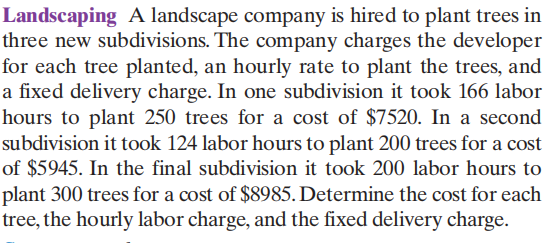### ¿Todavía tienes preguntas de matemáticas?

Pregunte a nuestros tutores expertos
Algebra
PreguntaA landscape company is hired to plant trees in three new subdivisions. The company charges the developer for each tree planted, an hourly rate to plant the trees, and a fixed delivery charge. In one subdivision it took $$166$$ labor hours to plant $$250$$ trees for a cost of $$\ 7520$$ . In a second subdivision it took $$124$$ labor hours to plant $$200$$ trees for a cost of $$\ 5945$$ . In the final subdivision it took $$200$$ labor hours to plant $$300$$ trees for a cost of $$\ 8985$$ . Determine the cost for each tree, the hourly labor charge, and the fixed delivery charge.

the cost of each tree: $$x = \19.95$$
the hourly labor cost: $$y = \13.75$$
delivery cost: $$C = \250$$